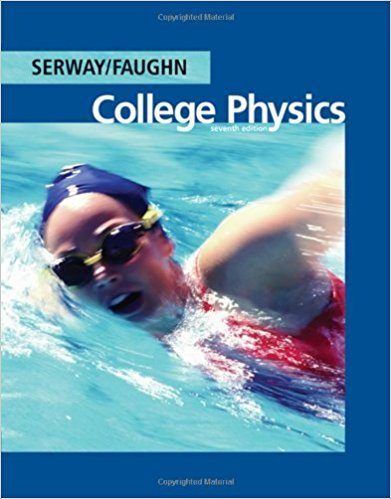×
Get Full Access to College Physics - 7 Edition - Chapter 18 - Problem 18.25
Get Full Access to College Physics - 7 Edition - Chapter 18 - Problem 18.25

×

# Calculate each of the unknown currents I1, I2, and I3 forISBN: 9780495113690 154

## Solution for problem 18.25 Chapter 18

College Physics | 7th Edition

• Textbook Solutions
• 2901 Step-by-step solutions solved by professors and subject experts
• Get 24/7 help from StudySoup virtual teaching assistantsCollege Physics | 7th Edition

4 5 1 238 Reviews
23
5
Problem 18.25

Calculate each of the unknown currents I1, I2, and I3 for the circuit of Figure P18.25.

Step-by-Step Solution:
Step 1 of 3

1/29/16 Todaywelearntheprincipal behind: Physics 205 (adding heat to get work Friday 1/29/16 18.5 1 law of thermodynamics Engines: over all change internal

Step 2 of 3

Step 3 of 3

##### ISBN: 9780495113690

Since the solution to 18.25 from 18 chapter was answered, more than 255 students have viewed the full step-by-step answer. This textbook survival guide was created for the textbook: College Physics, edition: 7. The answer to “Calculate each of the unknown currents I1, I2, and I3 for the circuit of Figure P18.25.” is broken down into a number of easy to follow steps, and 16 words. This full solution covers the following key subjects: calculate, Circuit, currents, figure, unknown. This expansive textbook survival guide covers 30 chapters, and 2135 solutions. The full step-by-step solution to problem: 18.25 from chapter: 18 was answered by , our top Physics solution expert on 11/15/17, 04:28PM. College Physics was written by and is associated to the ISBN: 9780495113690.

Unlock Textbook Solution